27 Applying Functions Repeatedly
 In:=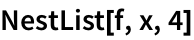Out=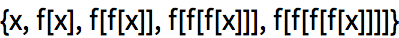Using Framed as the function makes it a little more obvious whats going on:
 In:=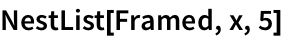Out=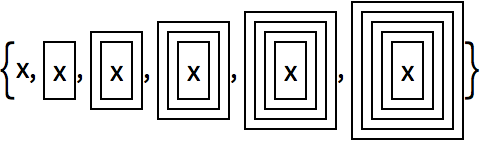This gives the final result of 5 levels of nesting:
 In:=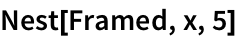Out=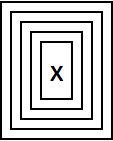Nestedly apply EdgeDetect to an image, first finding edges, then edges of edges and so on.
Nestedly do edge detection on an image:
 In:=Out=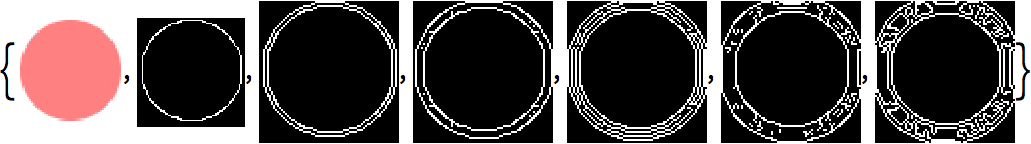In:=Out=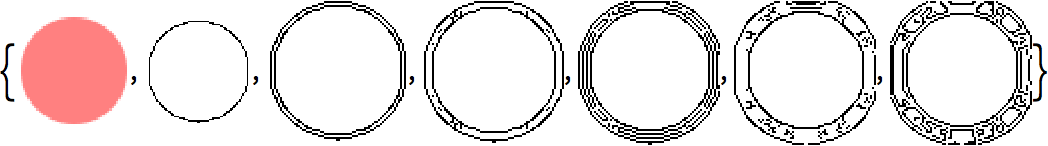In:=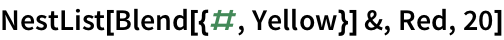Out=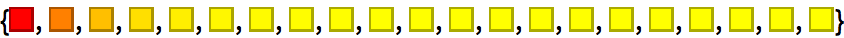If you successively apply a function that adds 1, you just get successive integers.
 In:=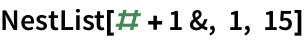Out=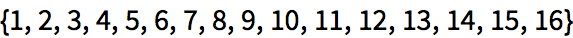Nestedly multiply by 2, getting powers of 2.
 In:=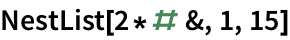Out=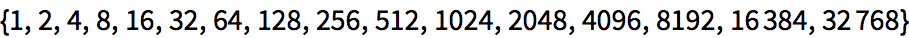In:=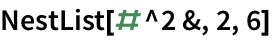Out=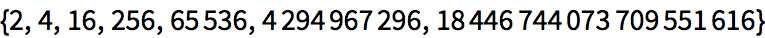Nestedly apply square root:
 In:=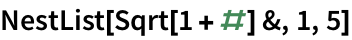Out=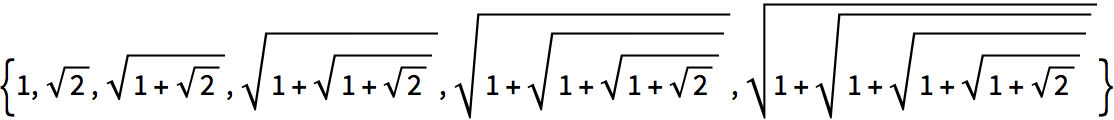In:=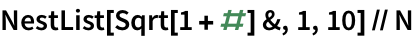Out=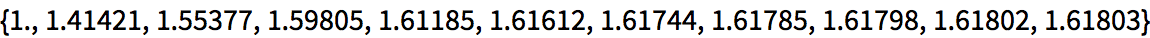RandomChoice randomly chooses from a list. You can use it to create a pure function that, say, randomly either adds +1 or 1.
Randomly add or subtract 1 at each step, starting from 0:
 In:=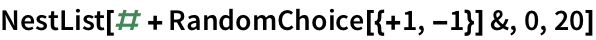Out=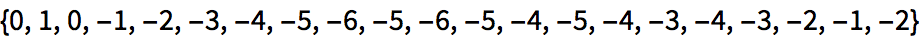In:=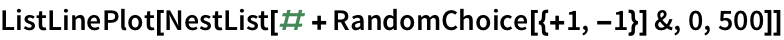Out=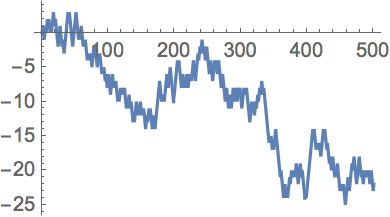So far, weve used NestList iterativelyeffectively to perform a chain of applications of a particular function. But you can also use it for recursion, in which the very pattern of applications of the function is itself nested.
This does a chain of applications of the function f:
 In:=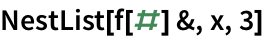Out=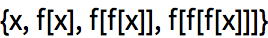The pattern of applications of f is more complicated here:
 In:=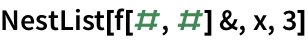Out=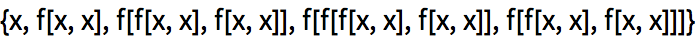In:=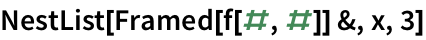Out=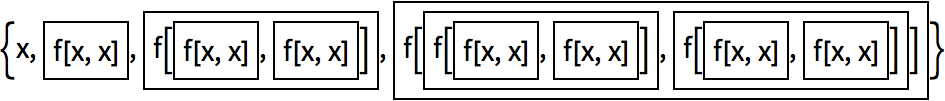The nested boxes are recursively combined in twos at each level:
 In:=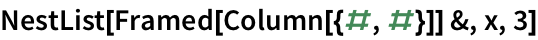Out=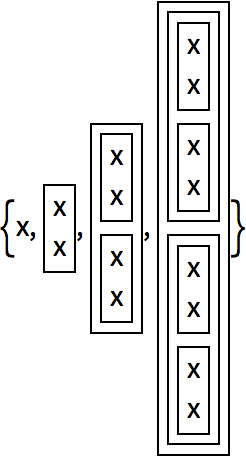In:=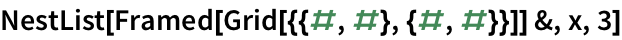Out=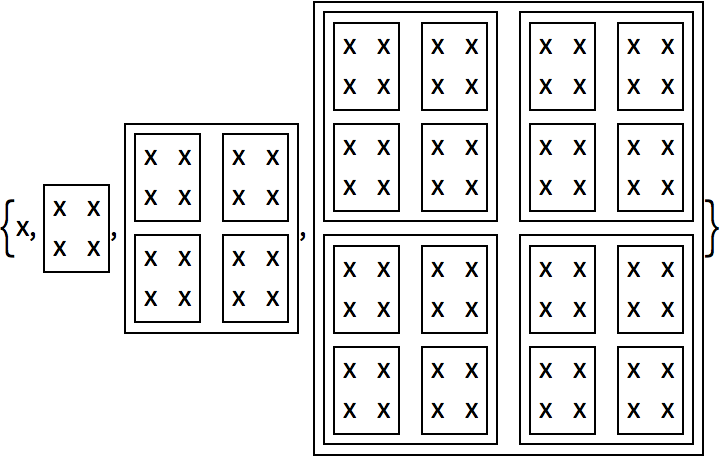In:=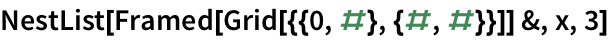Out=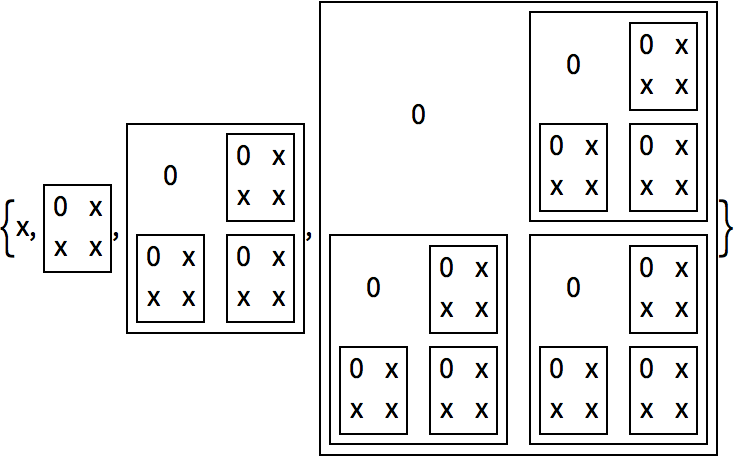In:=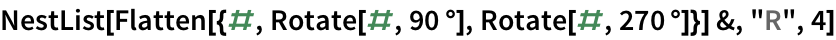Out=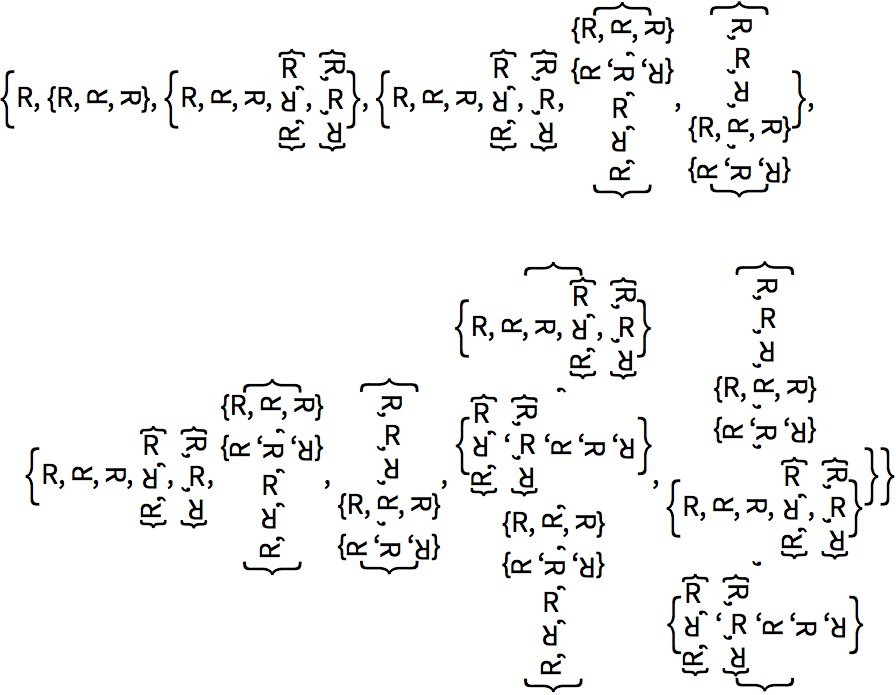In:=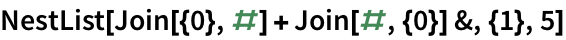Out=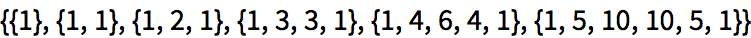In:=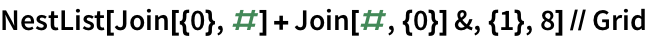Out=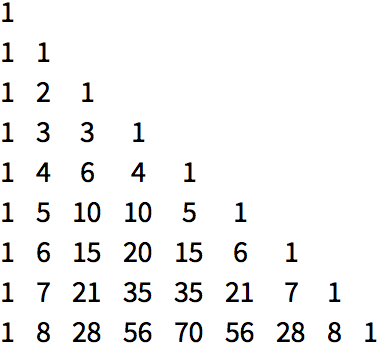Form a recursive structure with two functions f and g:
 In:=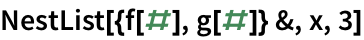Out=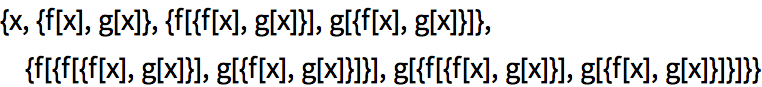Even if things are arranged in columns, its still quite difficult to understand the structure thats been created.
Arrange the recursive structure in columns:
 In:=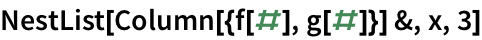Out=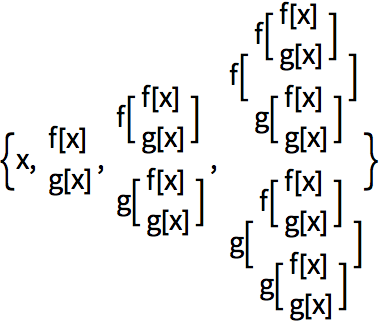NestGraph is basically like NestList, except that it makes a graph rather than a list. It repeatedly applies a function to determine what nodes a particular node should connect to. In this case, it produces a tree of nodes, making it clearer whats going on.
 In:=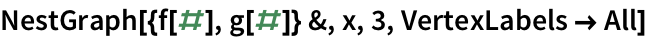Out=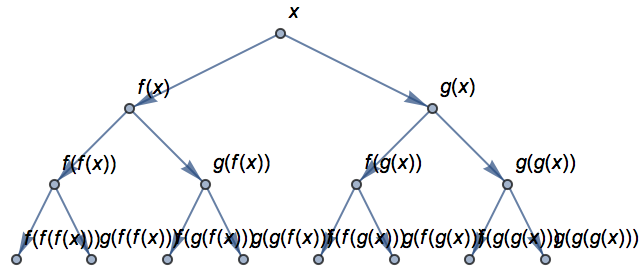Repeatedly apply a numerical function to form another tree structure:
 In:=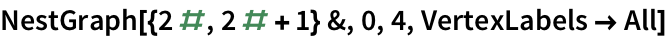Out=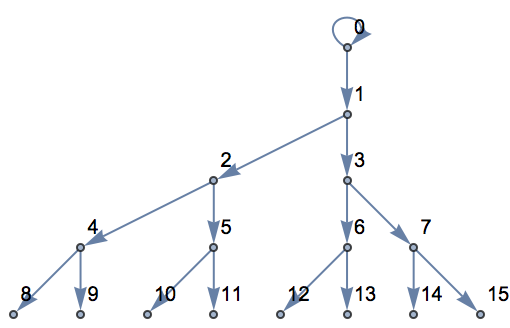You can use NestGraph to effectively crawl outward creating a network. As an example, we can repeatedly apply a function that for any country gives a list of countries that border it. The result is a network that connects bordering countries, here starting with Switzerland.
Crawl outward 2 steps from Switzerland, connecting each country to all those that border it:
 In:=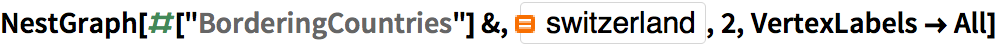Out=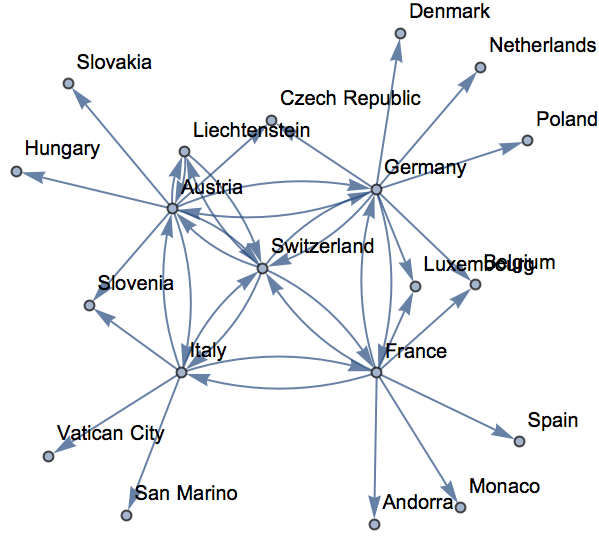Create a network of nearby words with respect to 1-letter changes:
 In:=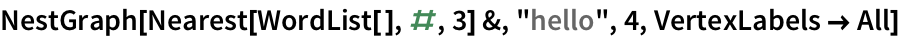Out=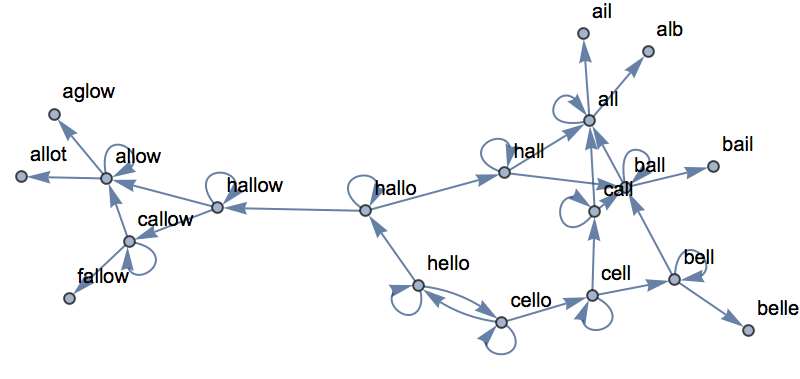27.1Make a list of the results of nesting Blur up to 10 times, starting with a rasterized size-30 X»
Expected output:
 Out[]=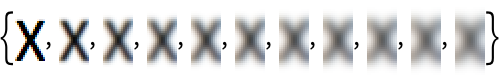Answer & check your solution
27.2Start with x, then make a list by nestedly applying Framed up to 10 times, using a random background color each time. »
Sample expected output:
 Out[]=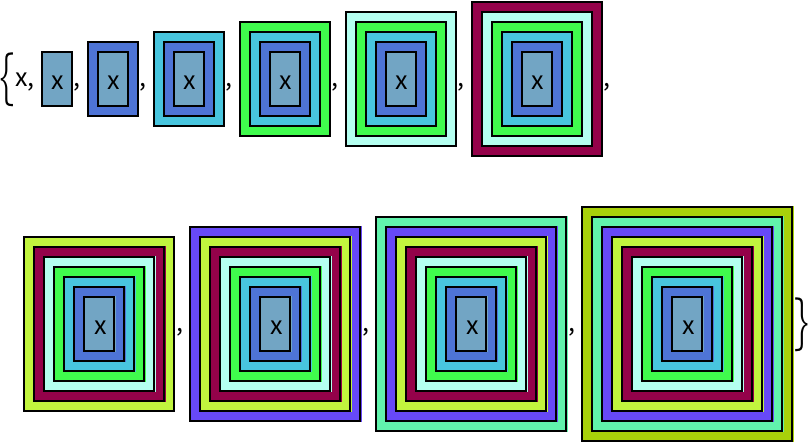Answer & check your solution
27.3Start with a size-50 A, then make a list of nestedly applying a frame and a random rotation 5 times. »
Sample expected output:
 Out[]=Answer & check your solution
27.4Make a line plot of 100 iterations of the logistic map iteration 4 #(1-#)&, starting from 0.2. »
Expected output:
 Out[]=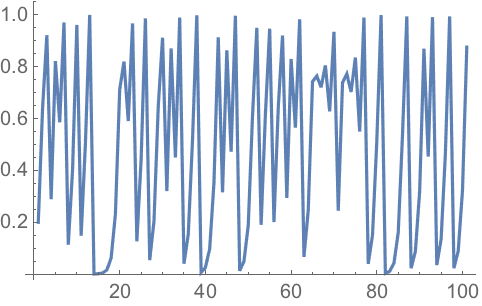Answer & check your solution
27.5Find the numerical value of the result from 30 iterations of 1+1/#& starting from 1. »
Expected output:
 Out[]=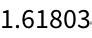Answer & check your solution
27.6Create a list of the first 10 powers of 3 (starting at 0) by nested multiplication. »
Expected output:
 Out[]=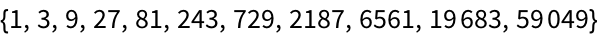Answer & check your solution
27.7Make a list of the result of nesting the (Newtons method) function (#+2/#)/2& up to 5 times starting from 1.0, and then subtract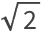from all the results. »
Expected output:
 Out[]=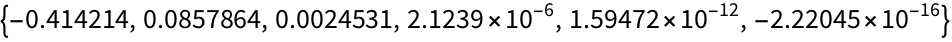Answer & check your solution
27.8Make graphics of a 1000-step 2D random walk which starts at {0, 0}, and in which at each step a pair of random numbers between 1 and +1 are added to the coordinates. »
Sample expected output:
 Out[]=Answer & check your solution
Expected output:
 Out[]=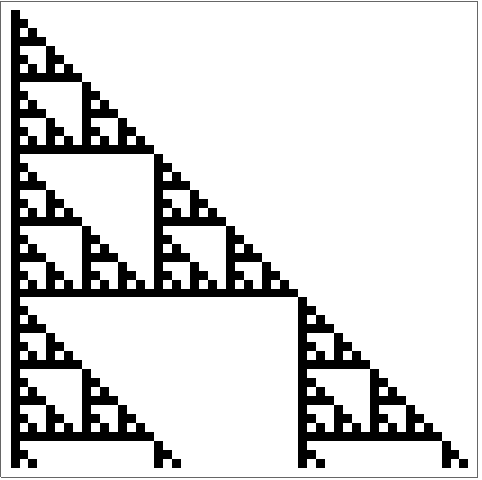Answer & check your solution
27.10Generate a graph by starting from 0, then nestedly 10 times connecting each node with value n to ones with values n+1 and 2n»
Expected output:
 Out[]=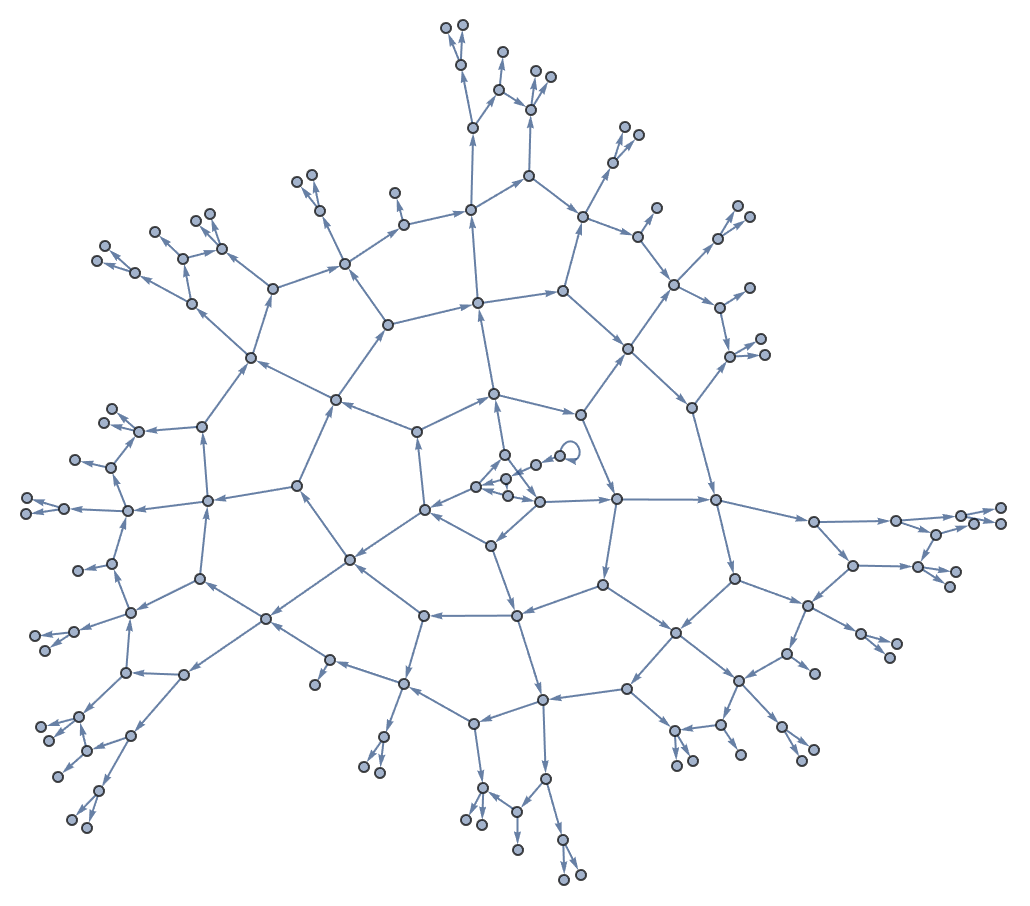Answer & check your solution
27.11Generate a graph obtained by nestedly finding bordering countries starting from the United States, and going 4 iterations. »
Expected output:
 Out[]=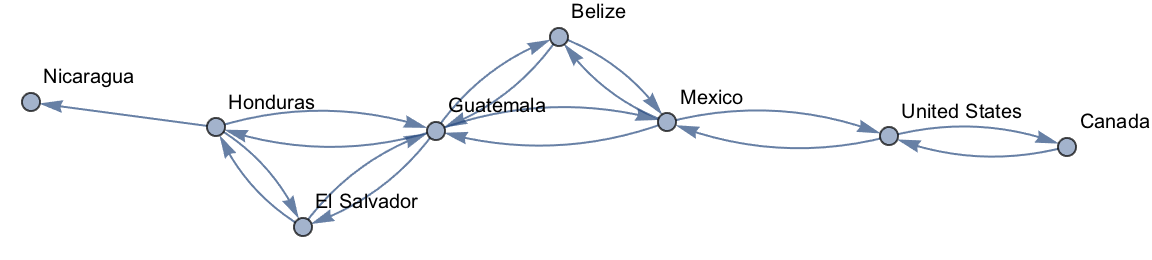Answer & check your solution
+27.1Make a line plot of 100 iterations of linear congruential function Mod[59#, 101]&, starting from 1. »
Expected output:
 Out[]=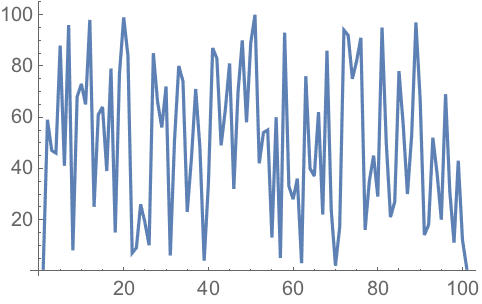Answer & check your solution
+27.2Create a list of 5 power towers of 2, i.e. 2^2^2...^2 n times, with n from 0 to 4. »
Expected output:
 Out[]=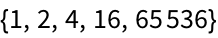Answer & check your solution
+27.3Create a list of 20 power towers of 1.2, i.e. 1.2^1.2^...^1.2 n times, with n from 0 to 19. »
Expected output:
 Out[]=Answer & check your solution
+27.4Generate a list of the numerical values obtained by up to 10 nestings of the function Sqrt[1+#]&»
Expected output:
 Out[]=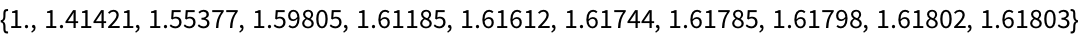Answer & check your solution
+27.5Make graphics of a 1000-step 3D random walk which starts at {0, 0, 0}, and in which at each step a triple of random numbers between 1 and +1 are added to the coordinates. »
Sample expected output:
 Out[]=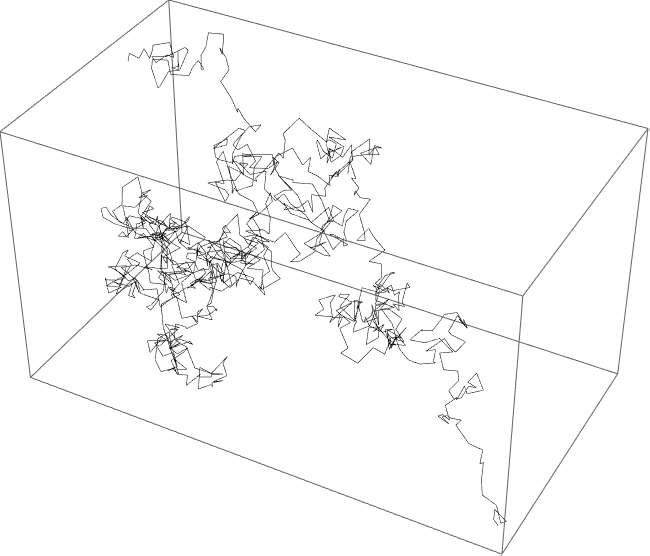Answer & check your solution
Whats the difference between iteration and recursion?
What is the relation between nesting, recursion and fractals?
What is Pascals triangle?
Is NestGraph like a web crawler?
Why do some but not all countries have arrows both ways in the bordering countries graph?
If NestGraph was run for enough steps, all countries would have arrows both ways, since if A borders B, then B borders A. But here were stopping after just 2 steps, so many of the reverse connections havent been reached.
Why use NestList for something like NestList[2*#&, 1, 15]?
You dont need to. You can just use Power, as in Table[2^n, {n, 0, 15}]. But its nice to see the sequence Plus, Times, Power arise from successive nesting (e.g. NestList[2+#&, 0, 15] is Table[2*n, {n, 0, 15}]).
Is there a way to keep applying a function until nothing is changing?